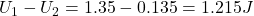## A particle (charge 7.5 µC) is released from rest at a point on the x axis, x = 10 cm. It begins to move due to the presence of a 2.0-µC char

Question

A particle (charge 7.5 µC) is released from rest at a point on the x axis, x = 10 cm. It begins to move due to the presence of a 2.0-µC charge which remains fixed at the origin. What is the kinetic energy of the particle at the instant it passes the point x = 1.0 m?

in progress 0
4 weeks 2021-08-19T12:54:56+00:00 1 Answers 0 views 0

1.215J

Explanation:

Using the law of energy conservation, the potential energy is equal to the kinetic energy of the particle during motion: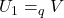#the V of the 2.0-µC is expressed as: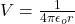We substitute the given values from the question:

##When the moving charge reaches 0.1meter the energy becomes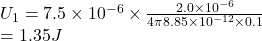#When the moving charge reaches 1.0 meter the energy becomes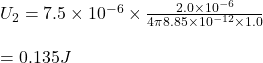Hence, the change in energy is